11: Efficiency in Numbers (II)

The most difficult part of an escapement drawing is the calculations for the verge circle radii, which is critical for the Pin Wheel escapement. Here there are seven circles (not drawn to scale).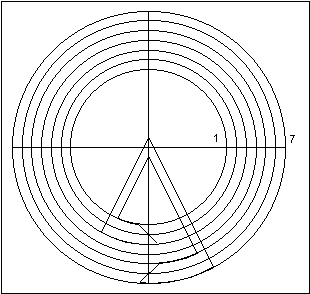If the 4th circle, between the pallets, has a radius of 6", the others must be calculated from this circle. If the escape wheel's pin has a diameter of 1/16 of an inch, or 0.0625", the gap between the two pallets must be greater than this, say 0.1": the radii of the 3rd and the 5th circles would be 5.95" and 6.05" respectively. The gap between the locking face of the entry pallet and the let-off corner of the exit pallet must be equal to the arc between two escape wheel pins (less the diameter of one pin and less the allowance for drop). If the escape wheel had fifteen pins and a radius of 3", its arc would be:

arc = 3 x (24ş x pi) / 180 = 1.26"
(because 24ş x 15 = 360ş), but you allow 1.5ş for drop:
arc = 3 x (22.5 x pi) / 180 = 1.18"
and for the pin diameter: arc = 1.18 - 0.0625 = 1.12" (to two decimal places).

The radius of the 1st circle is therefore:     6 - (1.12 / 2) = 5.44"
The radius of the 7th circle is 6.56". The radius of the 2nd circle is half way between the 1st and the 3rd:(5.95 + 5.44) / 2 = 5.70"
The radius of the 6th circle is half way between the 5th and the 7th:(6.05 + 6.56) / 2 = 6.30"

The radius of the 4th circle may appear to be arbitrary because it is not determined by the tooth span between the pallets, as it would be for the Graham, Recoil, and Brocot. It is determined only by the desired angle of pendulum swing.

Consider a pallet circle that was determined by a tooth span, such as the Graham escapement with the 15 tooth escape wheel in chapter 10. It had a tooth span of 5.5 teeth, so the angle between the pallets was: (5.5 / 15) x 360 = 132ş, half of which was 66ş. The escape circle radius was 3", so the pallet circle radius could be found by triangulation: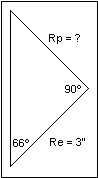tan (66) = Rp / Re
Rp = Re x tan (66) = 3 tan (66) = 6.74"
Continuing with the Graham in chapter 10, its escape wheel had a radius of 3", so the arc between two teeth was:
arc = (3" x 24ş x pi) / 180ş = 1.26"
The arc per beat (12ş): 1.26 / 2 = 0.63"
Allow 1.5ş for drop:
arc = (0.63 x 10.5ş) / 12ş = 0.55"
This is the thickness of the pallets.
Therefore, the entry pallet locking face has a pallet circle radius of:
6.74 + 0.27 = 7.01"
and the exit pallet locking face has a pallet circle radius of:
6.74 - 0.27 = 6.47".

Replacing the Pallets.
Suppose a British grandfather clock movement were brought for repair with no pallets. You determine by inspecting the 30 tooth escape wheel that the clock had a recoil escapement. The distance between the escape wheel bushing and the pallet bushing is 1.6". The escape wheel has a diameter of 2", so the escape circle's radius is 1". First, draw the pallet you want over an ideal Graham pallet and remove the latter.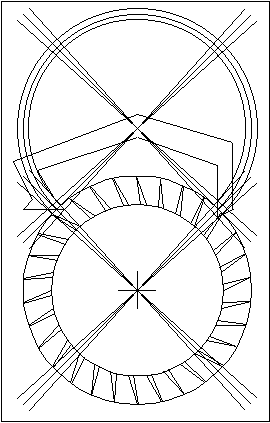Draw two triangles using this arrangement:
cos (A) = 1 / 1.6
A = acos (1 / 1.6) = 51.3ş
Use this to determine the tooth span:
30 x (2 x 51.3 / 360) = 8.553
so you should use a span of 8.5 teeth.To recalculate the angle A: (8.5 x 360) / (2 x 30) = A = 51ş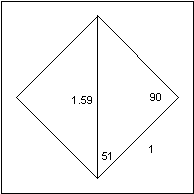Assume a small error in measurement:
cos (51) = 1 / h
h = 1 / cos (51) = 1.59"
The pallet angle is : 180 - 90 - 51 = 39ş
Rp = 1 x tan (51) = 1.23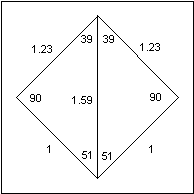By comparing this drawing with the one on the previous page, calculate the positions of the tips of the pallets. The inside tip of the entry pallet needs to be at a slightly smaller angle from vertical:
Angle = 39 - (3 x (1 / 1.23) = 36.6ş
r = 1.23 - ((2 x pi) / (4 x 30)) = 1.18"
Similarly for the exit pallet:
Angle = 36.6ş.
r = 1.23 + ((2 x pi) / (4 x 30)) = 1.28"

The rest of the pallet could be drawn any way you wish.To explain the numbers above, the escape wheel has an angle of 12ş between two teeth. A tooth will travel 6ş per beat. The pallet angle lines were divided into 3ş on either side. Since the pallet radius is 1.23 and the escape radius is 1", multiply the escape angle of 3ş by the ratio of the radii. Since the pallet radius is larger, the pallet arc is smaller than the escape wheel arc (which has an angle of 3ş).

For the radius calculation, decrease the pallet radius on the entry pallet by the amount of a quarter of the arc between two escape wheel teeth, and increase the pallet radius on the exit pallet similarly. This is only a close approximation because the change in the pallet circle's radius is assumed to be equal to the arc of the escape circle, but an arc is not a straight line.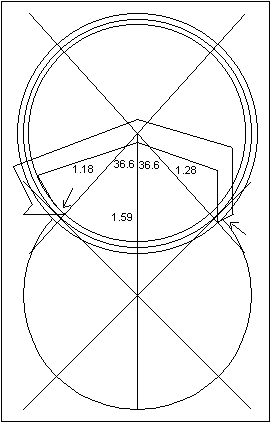Once the pallet blank is made, it must be fitted because there is no drop. By filing on the surfaces pointed to by the arrows, some inside and outside drop could be created.

When designing a Graham pallet, the issue becomes more complicated because of lock. I would suggest that the best way to design the pallets would be to make the blank with impulse faces that have angles relative to Fe smaller than 45ş, such as 25ş. When fitting the pallets to the clock, file first on the inside and outside surfaces to create enough inside and outside drop. Then file on the impulse face to increase the impulse angle until the lock has been reduced to about 1ş. To be practical in a manufacturing situation, the measurement deviations must be accounted for to keep rejects statistically below 5%. The need to account for these deviations means that a compromise situation arises because fitting each verge by hand would interfere with mass production and economies of scale.

Fitting a Brocot pallet requires the calculation of the pallet circle's radius. If the escape wheel rotates 6ş per beat, and its radius were 1", the arc would be:
arc = (1 x 6 x pi) / 180 = 0.105"
I suggest 1.5ş of drop, so the radius of the pallet's circle should be:
r = (1 x 4.5 x pi) / 180 = 0.08"
Using the recoil example, the Brocot pallet should be positioned such that the point where the pallet releases the escape tooth should have a pallet circle radius of 1.18" and an angle of 36.6ş from vertical for the entry pallet, and a radius of 1.28" and an angle of 36.6ş for the exit pallet. Then the lock and drop could be further adjusted by raising or lowering the pallets, or by changing the gap between the pallets.

By studying the above example, you could see how you could use one type of escapement for the calculations, and then apply them to the type of escapement you want to create. A step-by-step process makes the calculations easier.